# Quora Insincere Questions Classification - Part 1/2

Side note : this is the first part of two, see the conclusion for the next part.

## Context

An existential problem for any major website today is how to handle toxic and divisive content. Quora wants to tackle this problem head-on to keep their platform a place where users can feel safe sharing their knowledge with the world.

Quora is a platform that empowers people to learn from each other. On Quora, people can ask questions and connect with others who contribute unique insights and quality answers. A key challenge is to weed out insincere questions – those founded upon false premises, or that intend to make a statement rather than look for helpful answers.

In this competition, Kagglers will develop models that identify and flag insincere questions. To date, Quora has employed both machine learning and manual review to address this problem. With your help, they can develop more scalable methods to detect toxic and misleading content.

Here’s your chance to combat online trolls at scale. Help Quora uphold their policy of “Be Nice, Be Respectful” and continue to be a place for sharing and growing the world’s knowledge.

## Goal

Predict whether a question asked on Quora is sincere or not

An insincere question is defined as a question intended to make a statement rather than look for helpful answers. Some characteristics that can signify that a question is insincere:

• Has a non-neutral tone
• Is disparaging or inflammatory
• Isn’t grounded in reality
• Uses sexual content (incest, bestiality, pedophilia) for shock value, and not to seek genuine answers

## Dataset

The training data includes the question that was asked, and whether it was identified as insincere (target = 1). The ground-truth labels contain some amount of noise: they are not guaranteed to be perfect.

Note that the distribution of questions in the dataset should not be taken to be representative of the distribution of questions asked on Quora. This is, in part, because of the combination of sampling procedures and sanitization measures that have been applied to the final dataset.

# Exploratory Data Analysis

Libraries import

``````import warnings
warnings.filterwarnings("ignore")
``````
``````import numpy as np
import pandas as pd

import seaborn as sns
import matplotlib.pyplot as plt
%matplotlib inline
``````
``````from nltk.tokenize import word_tokenize
from nltk.corpus import stopwords
from nltk.stem import PorterStemmer

from string import punctuation

from sklearn.model_selection import train_test_split
from sklearn.pipeline import Pipeline
from sklearn.linear_model import LogisticRegression
from sklearn.feature_extraction.text import CountVectorizer, TfidfVectorizer
from sklearn.metrics import f1_score, classification_report
``````
``````from xgboost import XGBClassifier
import lightgbm as lgb
``````

File descriptions

• train.csv - the training set
• test.csv - the test set
• sample_submission.csv - A sample submission in the correct format
• enbeddings/ - (see below)
``````df = pd.read_csv("../input/quora-insincere-questions-classification/train.csv")
``````
qid question_text target
0 00002165364db923c7e6 How did Quebec nationalists see their province... 0
1 000032939017120e6e44 Do you have an adopted dog, how would you enco... 0
2 0000412ca6e4628ce2cf Why does velocity affect time? Does velocity a... 0
3 000042bf85aa498cd78e How did Otto von Guericke used the Magdeburg h... 0
4 0000455dfa3e01eae3af Can I convert montra helicon D to a mountain b... 0

Data fields

• qid - unique question identifier
• question_text - Quora question text
• target - a question labeled “insincere” has a value of 1, otherwise 0
``````pd.read_csv("../input/quora-insincere-questions-classification/test.csv").head()
``````
qid question_text
0 0000163e3ea7c7a74cd7 Why do so many women become so rude and arroga...
1 00002bd4fb5d505b9161 When should I apply for RV college of engineer...
2 00007756b4a147d2b0b3 What is it really like to be a nurse practitio...
3 000086e4b7e1c7146103 Who are entrepreneurs?
4 0000c4c3fbe8785a3090 Is education really making good people nowadays?

## Basic infos and analysis of the target

``````df.info()
``````
``````<class 'pandas.core.frame.DataFrame'>
RangeIndex: 1306122 entries, 0 to 1306121
Data columns (total 3 columns):
qid              1306122 non-null object
question_text    1306122 non-null object
target           1306122 non-null int64
dtypes: int64(1), object(2)
memory usage: 29.9+ MB
``````

No Nan and no duplicated line :

``````df.duplicated().sum()
``````
``````0
``````

Target analysis

``````df.target.value_counts()
``````
``````0    1225312
1      80810
Name: target, dtype: int64
``````
``````df.target.describe()
``````
``````count    1.306122e+06
mean     6.187018e-02
std      2.409197e-01
min      0.000000e+00
25%      0.000000e+00
50%      0.000000e+00
75%      0.000000e+00
max      1.000000e+00
Name: target, dtype: float64
``````
``````plt.figure(figsize=(5, 4))
sns.countplot(x='target', data=df)
plt.title('Reparition of question by sincerity (insincere = 1)');
``````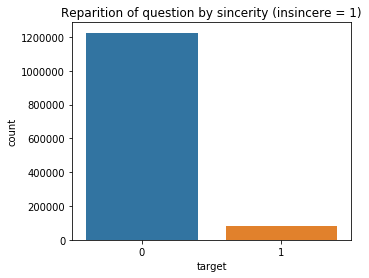``````print(f'There are {df.target.sum() / df.shape * 100 :.1f}% of insincere questions, which make the dataset highly unbalanced.')
``````
``````There are 6.2% of insincere questions, which make the dataset highly unbalanced.
``````

## Word clouds

Generally, though, data scientists don’t think much of word clouds, in large part because the placement of the words doesn’t mean anything other than “here’s some space where I was able to fit a word.” Anyway, clouds can come handy to have a frist insight of the most common words…

Word clouds (also known as text clouds or tag clouds) work in a simple way: the more a specific word appears in a source of textual data (such as a speech, blog post, or database), the bigger and bolder it appears in the word cloud.

``````from wordcloud import WordCloud, STOPWORDS
stopwords = set(STOPWORDS)
``````
``````print('Word cloud image generated from sincere questions')
sincere_wordcloud = WordCloud(width=600, height=400, background_color ='white', min_font_size = 10).generate(str(df[df["target"] == 0]["question_text"]))
#Positive Word cloud
plt.figure(figsize=(15,6), facecolor=None)
plt.imshow(sincere_wordcloud)
plt.axis("off")
plt.show();
``````
``````Word cloud image generated from sincere questions
````````````print('Word cloud image generated from INsincere questions')
insincere_wordcloud = WordCloud(width=600, height=400, background_color ='white', min_font_size = 10).generate(str(df[df["target"] == 1]["question_text"]))
#Positive Word cloud
plt.figure(figsize=(15,6), facecolor=None)
plt.imshow(insincere_wordcloud)
plt.axis("off")
plt.show();
``````
``````Word cloud image generated from INsincere questions
``````## Statistics form the question texts

The process of converting data to something a computer can understand is referred to as pre-processing. One of the major forms of pre-processing is to filter out useless data. In natural language processing, useless words (data), are referred to as stop words.

``````# if needed
``````

Stop Words: A stop word is a commonly used word (such as “the”, “a”, “an”, “in”) that a search engine has been programmed to ignore, both when indexing entries for searching and when retrieving them as the result of a search query.

We would not want these words taking up space in our database, or taking up valuable processing time. For this, we can remove them easily, by storing a list of words that you consider to be stop words. NLTK(Natural Language Toolkit) in python has a list of stopwords stored in 16 different languages. You can find them in the nltk_data directory.

``````import nltk
from nltk.corpus import stopwords
stop_words = set(stopwords.words('english'))
stop_words
``````
``````{'a',
'above',
'after',
'again',
'against',
'ain',
'all',
'am',
'an',
'and',
'any',
'are',
'aren',
"aren't",
'as',
'at',
'be',
'because',
'been',
'before',
'being',
'below',
'between',
'both',
'but',
'by',
'can',
'couldn',
"couldn't",
'd',
'did',
'didn',
"didn't",
'do',
'does',
'doesn',
"doesn't",
'doing',
'don',
"don't",
'down',
'during',
'each',
'few',
'for',
'from',
'further',
'has',
'hasn',
"hasn't",
'have',
'haven',
"haven't",
'having',
'he',
'her',
'here',
'hers',
'herself',
'him',
'himself',
'his',
'how',
'i',
'if',
'in',
'into',
'is',
'isn',
"isn't",
'it',
"it's",
'its',
'itself',
'just',
'll',
'm',
'ma',
'me',
'mightn',
"mightn't",
'more',
'most',
'mustn',
"mustn't",
'my',
'myself',
'needn',
"needn't",
'no',
'nor',
'not',
'now',
'o',
'of',
'off',
'on',
'once',
'only',
'or',
'other',
'our',
'ours',
'ourselves',
'out',
'over',
'own',
're',
's',
'same',
'shan',
"shan't",
'she',
"she's",
'should',
"should've",
'shouldn',
"shouldn't",
'so',
'some',
'such',
't',
'than',
'that',
"that'll",
'the',
'their',
'theirs',
'them',
'themselves',
'then',
'there',
'these',
'they',
'this',
'those',
'through',
'to',
'too',
'under',
'until',
'up',
've',
'very',
'was',
'wasn',
"wasn't",
'we',
'were',
'weren',
"weren't",
'what',
'when',
'where',
'which',
'while',
'who',
'whom',
'why',
'will',
'with',
'won',
"won't",
'wouldn',
"wouldn't",
'y',
'you',
"you'd",
"you'll",
"you're",
"you've",
'your',
'yours',
'yourself',
'yourselves'}
``````
``````def create_features(df_):
"""Retrieve from the text column the nb of : words, unique words, characters, stopwords,
punctuations, upper/lower case char, title..."""

df_["nb_words"] = df_["question_text"].apply(lambda x: len(x.split()))
df_["nb_unique_words"] = df_["question_text"].apply(lambda x: len(set(str(x).split())))
df_["nb_chars"] = df["question_text"].apply(lambda x: len(str(x)))
df_["nb_stopwords"] = df_["question_text"].apply(lambda x : len([nw for nw in str(x).split() if nw.lower() in stop_words]))
df_["nb_punctuation"] = df_["question_text"].apply(lambda x : len([np for np in str(x) if np in punctuation]))
df_["nb_uppercase"] = df_["question_text"].apply(lambda x : len([nu for nu in str(x).split() if nu.isupper()]))
df_["nb_lowercase"] = df_["question_text"].apply(lambda x : len([nl for nl in str(x).split() if nl.islower()]))
df_["nb_title"] = df_["question_text"].apply(lambda x : len([nl for nl in str(x).split() if nl.istitle()]))
return df_
``````
``````df = create_features(df)
df.sample(2)
``````
qid question_text target nb_words nb_unique_words nb_chars nb_stopwords nb_punctuation nb_uppercase nb_lowercase nb_title
685172 86319861df6a171eced7 What are some of the least economically develo... 0 9 9 60 5 1 0 8 1
302872 3b50058a0796f933924b Who invented ASMR? 0 3 3 18 1 1 1 1 1

Let’s take a sample - because the data set is quite huge when run locally on a single node - and visualize pair plots :

``````num_feat = ['nb_words', 'nb_unique_words', 'nb_chars', 'nb_stopwords', \
'nb_punctuation', 'nb_uppercase', 'nb_lowercase', 'nb_title', 'target']
# side note : remove target if needed later

df_sample = df[num_feat].sample(n=round(df.shape/6), random_state=42)

plt.figure(figsize=(16,10))
sns.pairplot(data=df_sample, hue='target')
plt.show()
``````
``````<Figure size 1152x720 with 0 Axes>
``````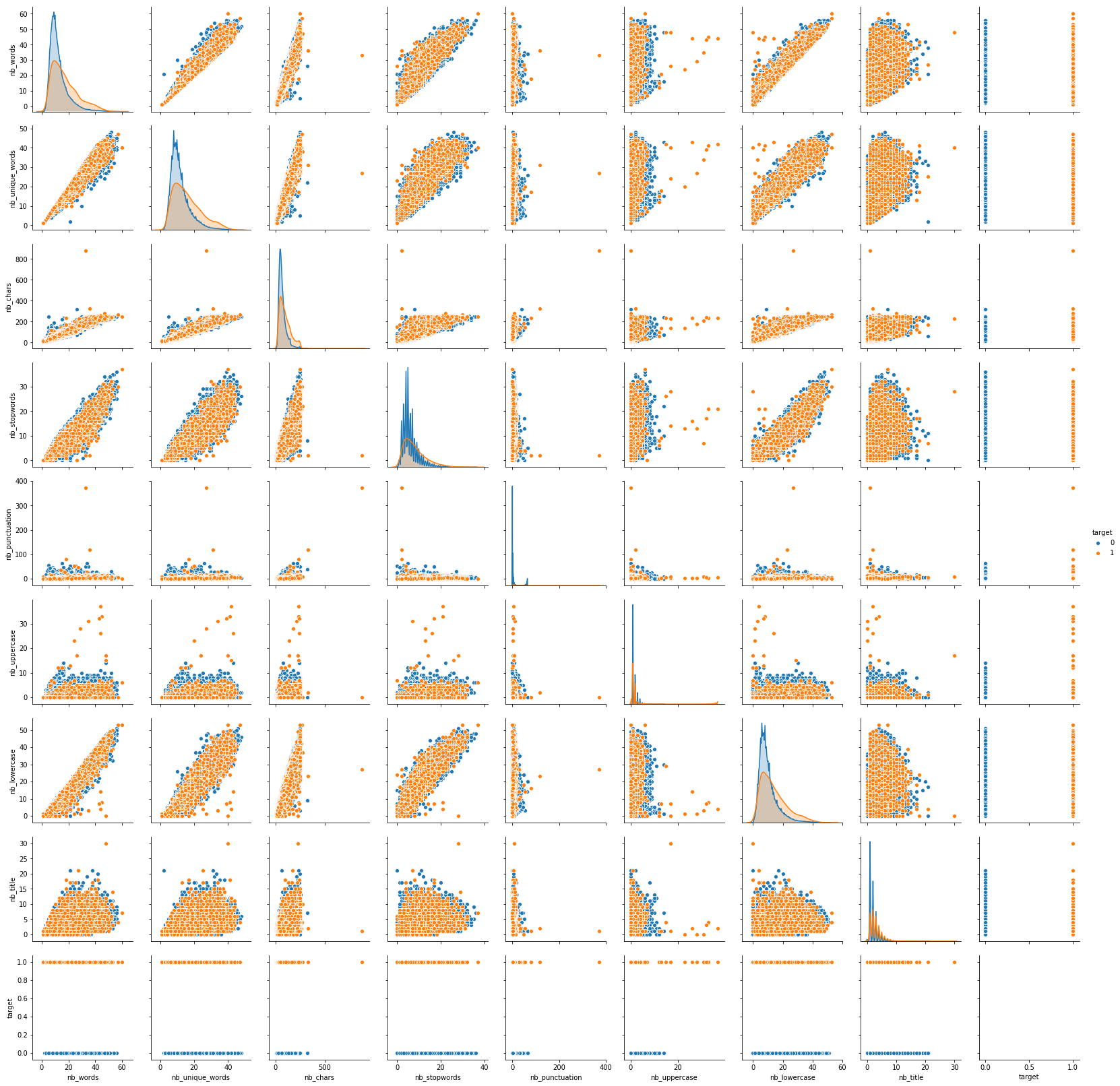Basic stats comparison :

``````df_sample[df_sample['target'] == 0].describe()
``````
nb_words nb_unique_words nb_chars nb_stopwords nb_punctuation nb_uppercase nb_lowercase nb_title target
count 204532.000000 204532.000000 204532.000000 204532.000000 204532.000000 204532.000000 204532.000000 204532.000000 204532.0
mean 12.509334 11.880190 68.885475 6.043426 1.706897 0.459860 10.035466 2.062655 0.0
std 6.751813 5.781951 36.732624 3.620446 1.545802 0.842419 6.172322 1.431942 0.0
min 2.000000 2.000000 10.000000 0.000000 0.000000 0.000000 0.000000 0.000000 0.0
25% 8.000000 8.000000 44.000000 4.000000 1.000000 0.000000 6.000000 1.000000 0.0
50% 11.000000 10.000000 59.000000 5.000000 1.000000 0.000000 8.000000 2.000000 0.0
75% 15.000000 14.000000 83.000000 7.000000 2.000000 1.000000 12.000000 3.000000 0.0
max 56.000000 48.000000 319.000000 36.000000 65.000000 14.000000 51.000000 21.000000 0.0
``````df_sample[df_sample['target'] == 1].describe()
``````
nb_words nb_unique_words nb_chars nb_stopwords nb_punctuation nb_uppercase nb_lowercase nb_title target
count 13155.000000 13155.000000 13155.000000 13155.000000 13155.000000 13155.000000 13155.000000 13155.000000 13155.0
mean 17.338502 16.073280 98.295325 8.063398 2.382516 0.339187 13.960775 2.973014 1.0
std 9.697397 8.224487 55.799960 5.056734 3.991050 1.029390 8.770353 1.992830 0.0
min 1.000000 1.000000 7.000000 0.000000 0.000000 0.000000 0.000000 0.000000 1.0
25% 10.000000 10.000000 54.000000 4.000000 1.000000 0.000000 7.000000 2.000000 1.0
50% 15.000000 14.000000 86.000000 7.000000 2.000000 0.000000 12.000000 3.000000 1.0
75% 23.000000 21.000000 130.000000 11.000000 3.000000 0.000000 19.000000 4.000000 1.0
max 60.000000 47.000000 878.000000 37.000000 372.000000 37.000000 53.000000 30.000000 1.0

Generally speaking, insincere questions are written with more words.

Now with a focus on the distributions, because there is a difference in the spikes between sincere and insincre questions.

``````plt.figure(figsize=(10,10))
plt.subplot(331)

i=0
for c in num_feat:
plt.subplot(3, 3, i+1)
i += 1
plt.title(c)

plt.show()
``````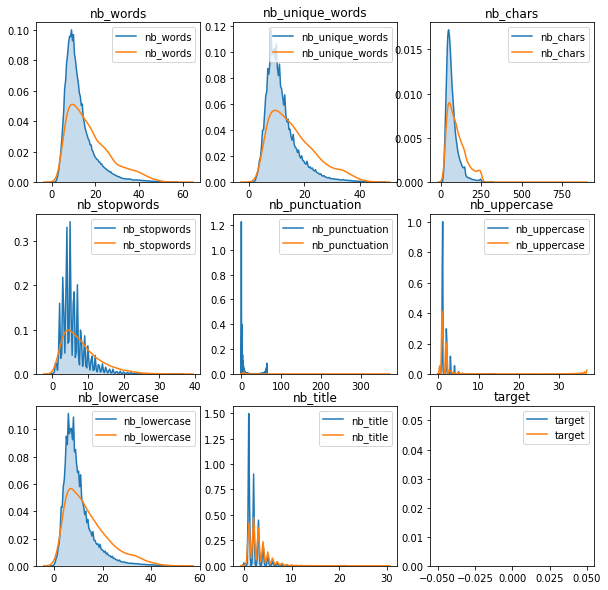Same conclusion here than shown with stats

Obviously, many of these indicators are highly correlated each other but not towards the target :

``````sns.set(style="white")

# Compute the correlation matrix
corr = df_sample[num_feat].corr()

# Generate a mask for the upper triangle

# Set up the matplotlib figure
f, ax = plt.subplots(figsize=(7, 6))

# Generate a custom diverging colormap
cmap = sns.diverging_palette(220, 10, as_cmap=True)

# Draw the heatmap with the mask and correct aspect ratio
square=True, linewidths=.5, annot=True, cbar_kws={"shrink": .5})
``````
``````<matplotlib.axes._subplots.AxesSubplot at 0x7f0d99b5eba8>
``````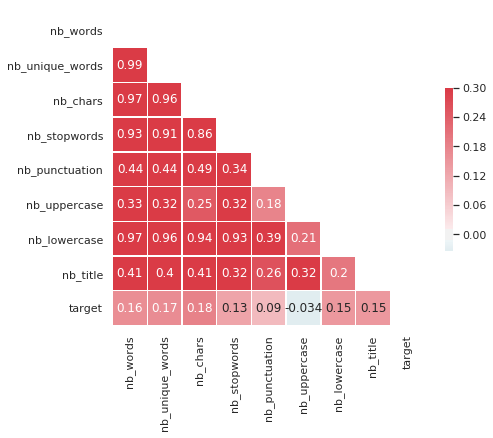What are the most frequent words for each type of question ?

``````class Vocabulary(object):
# credits : Shankar G see https://www.kaggle.com/kaosmonkey/visualize-sincere-vs-insincere-words

def __init__(self):
self.vocab = {}
self.STOPWORDS = set()
self.STOPWORDS = set(stopwords.words('english'))

def build_vocab(self, lines):
for line in lines:
for word in line.split(' '):
word = word.lower()
if (word in self.STOPWORDS):
continue
if (word not in self.vocab):
self.vocab[word] = 0
self.vocab[word] +=1

def generate_ngrams(text, n_gram=1):
"""arg: text, n_gram"""
token = [token for token in text.lower().split(" ") if token != "" if token not in STOPWORDS]
ngrams = zip(*[token[i:] for i in range(n_gram)])
return [" ".join(ngram) for ngram in ngrams]

def horizontal_bar_chart(df, color):
trace = go.Bar(
y=df["word"].values[::-1],
x=df["wordcount"].values[::-1],
showlegend=False,
orientation = 'h',
marker=dict(
color=color,
),
)
return trace
``````
``````sincere_vocab = Vocabulary()
sincere_vocab.build_vocab(df[df['target'] == 0]['question_text'])
sincere_vocabulary = sorted(sincere_vocab.vocab.items(), reverse=True, key=lambda kv: kv)

df_sincere_vocab = pd.DataFrame(sincere_vocabulary, columns=['word_sincere', 'frequency'])
sns.barplot(y='word_sincere', x='frequency', data=df_sincere_vocab[:20])
``````
``````<matplotlib.axes._subplots.AxesSubplot at 0x7f0d99d79ac8>
``````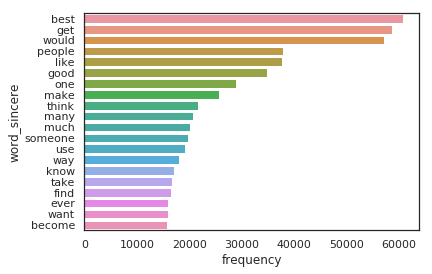``````insincere_vocab = Vocabulary()
insincere_vocab.build_vocab(df[df['target'] == 1]['question_text'])
insincere_vocabulary = sorted(insincere_vocab.vocab.items(), reverse=True, key=lambda kv: kv)

df_insincere_vocab = pd.DataFrame(insincere_vocabulary, columns=['word_insincere', 'frequency'])
sns.barplot(y='word_insincere', x='frequency', data=df_insincere_vocab[:20])
``````
``````<matplotlib.axes._subplots.AxesSubplot at 0x7f0d86abfa58>
``````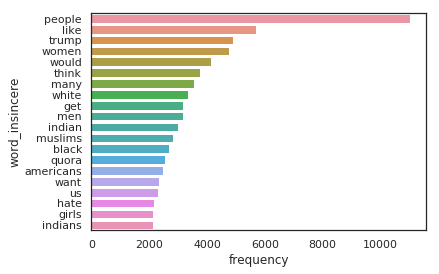As we can clearly see there are certain words (swear words, discriminatory words based on race, political figures etc) that show up a lot in insincere sentences.

# Text processing & model training

## Metric : F-score

The most appropriated metric is F1-score. Explanation from Wikipedia:

“In statistical analysis of binary classification, the F1 score (also F-score or F-measure) is a measure of a test’s accuracy. It considers both the precision p and the recall r of the test to compute the score: p is the number of correct positive results divided by the number of all positive results returned by the classifier, and r is the number of correct positive results divided by the number of all relevant samples (all samples that should have been identified as positive). The F1 score is the harmonic mean of the precision and recall, where an F1 score reaches its best value at 1 (perfect precision and recall) and worst at 0.”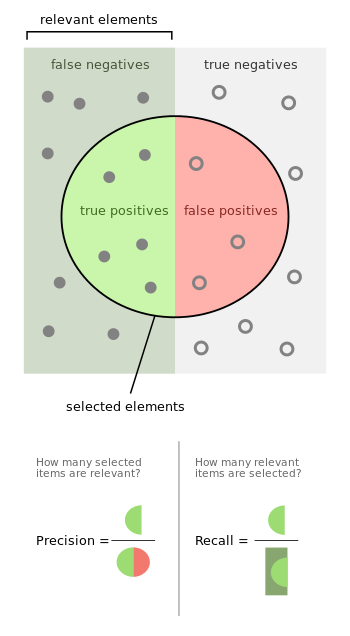we’ll also use a confusion matrix the most import part is the recall for insincere questions (class 1), because if the recall isn’t good enough, the f1-score won’t satisfying either. Here are mathematical definitions :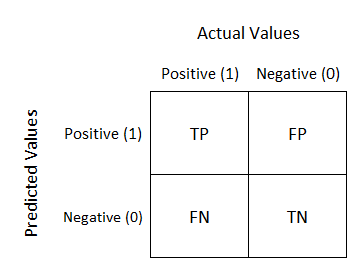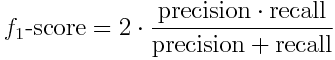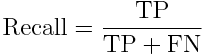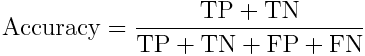``````def get_fscore_matrix(fitted_clf, model_name):
print(model_name, ' :')

# get classes predictions for the classification report
y_train_pred, y_pred = fitted_clf.predict(X_train), fitted_clf.predict(X_test)
print(classification_report(y_test, y_pred), '\n') # target_names=y

# computes probabilities keep the ones for the positive outcome only
print(f'F1-score = {f1_score(y_test, y_pred):.2f}')
``````

## Text processing

``````# if needed the first time
# import nltk
``````

Process : - tokenization - keeping only alphanumeriacl characters - removing stop words (punctuation etc…) - stemming or lemmatization.

source : blog.bitext.com

Tokenization : Given a character sequence and a defined document unit, tokenization is the task of chopping it up into pieces, called tokens , perhaps at the same time throwing away certain characters, such as punctuation

Stemming vs. lemmatization : The aim of both processes is the same: reducing the inflectional forms of each word into a common base or root.

Stemming algorithms work by cutting off the end or the beginning of the word, taking into account a list of common prefixes and suffixes that can be found in an inflected word. This indiscriminate cutting can be successful in some occasions, but not always, and that is why we affirm that this approach presents some limitations.

Lemmatization, on the other hand, takes into consideration the morphological analysis of the words. To do so, it is necessary to have detailed dictionaries which the algorithm can look through to link the form back to its lemma.

``````df = df[['question_text', 'target']]

def text_processing(local_df):
""" return the dataframe with tokens stemmetized without numerical values & stopwords """
stemmer = PorterStemmer()
# Perform preprocessing
local_df['txt_processed'] = local_df['question_text'].apply(lambda df: word_tokenize(df))
local_df['txt_processed'] = local_df['txt_processed'].apply(lambda x: [item for item in x if item.isalpha()])
local_df['txt_processed'] = local_df['txt_processed'].apply(lambda x: [item for item in x if item not in stop_words])
local_df['txt_processed'] = local_df['txt_processed'].apply(lambda x: [stemmer.stem(item) for item in x])
return local_df
``````
``````df = text_processing(df)
df.tail(2)
``````
question_text target txt_processed
1306120 How can one start a research project based on ... 0 [how, one, start, research, project, base, bio...
1306121 Who wins in a battle between a Wolverine and a... 0 [who, win, battl, wolverin, puma]

## First method : text similarity using TF-IDF

``````vectorizer = TfidfVectorizer(lowercase=False, analyzer=lambda x: x, min_df=0.01, max_df=0.999)
# min_df & max_df param added for less memory usage

tf_idf = vectorizer.fit_transform(df['txt_processed']).toarray()
``````
Do I If Is are becom best better book can ... where whi which who will without work world would year
0 0.000000 0.000000 0.0 0.0 0.0 0.0 0.0 0.0 0.0 0.00000 ... 0.0 0.000000 0.0 0.0 0.0 0.0 0.0 0.0 0.000000 0.0
1 0.606073 0.000000 0.0 0.0 0.0 0.0 0.0 0.0 0.0 0.00000 ... 0.0 0.000000 0.0 0.0 0.0 0.0 0.0 0.0 0.554503 0.0
2 0.000000 0.000000 0.0 0.0 0.0 0.0 0.0 0.0 0.0 0.00000 ... 0.0 0.416315 0.0 0.0 0.0 0.0 0.0 0.0 0.000000 0.0
3 0.000000 0.000000 0.0 0.0 0.0 0.0 0.0 0.0 0.0 0.00000 ... 0.0 0.000000 0.0 0.0 0.0 0.0 0.0 0.0 0.000000 0.0
4 0.000000 0.360439 0.0 0.0 0.0 0.0 0.0 0.0 0.0 0.56217 ... 0.0 0.000000 0.0 0.0 0.0 0.0 0.0 0.0 0.000000 0.0

5 rows × 76 columns

``````# Split the data
X_train, X_test, y_train, y_test = train_test_split(tf_idf, df['target'], test_size=0.2, random_state=42)
``````

XGBoost Classifier without weigths

``````model = XGBClassifier(objective="binary:logistic")
model.fit(X_train, y_train)
get_fscore_matrix(model, 'XGB Clf withOUT weights')
``````
``````XGB Clf withOUT weights  :
precision    recall  f1-score   support

0       0.94      1.00      0.97    245369
1       0.61      0.05      0.10     15856

micro avg       0.94      0.94      0.94    261225
macro avg       0.78      0.53      0.53    261225
weighted avg       0.92      0.94      0.92    261225

F1-score = 0.10
``````

XGBoost Classifier with weigths

``````ratio = ((len(y_train) - y_train.sum()) - y_train.sum()) / y_train.sum()
ratio
``````
``````14.086722911598978
``````
``````model = XGBClassifier(objective="binary:logistic", scale_pos_weight=ratio)
model.fit(X_train, y_train)
get_fscore_matrix(model, 'XGB Clf WITH weights')
``````
``````XGB Clf WITH weights  :
precision    recall  f1-score   support

0       0.98      0.81      0.89    245369
1       0.19      0.68      0.30     15856

micro avg       0.81      0.81      0.81    261225
macro avg       0.58      0.75      0.59    261225
weighted avg       0.93      0.81      0.85    261225

F1-score = 0.30
``````

Now LGBM with weights

``````model = lgb.LGBMClassifier(n_jobs = -1, class_weight={0:y_train.sum(), 1:len(y_train) - y_train.sum()})
model.fit(X_train, y_train)
get_fscore_matrix(model, 'LGBM weighted')
``````
``````LGBM weighted  :
precision    recall  f1-score   support

0       0.98      0.73      0.84    245369
1       0.16      0.80      0.26     15856

micro avg       0.73      0.73      0.73    261225
macro avg       0.57      0.76      0.55    261225
weighted avg       0.93      0.73      0.80    261225

F1-score = 0.26
``````

LogisticRegression

``````model = LogisticRegression(class_weight={0:y_train.sum(), 1:len(y_train) - y_train.sum()}, C=0.5, max_iter=100, n_jobs=-1)
model.fit(X_train, y_train)
get_fscore_matrix(model, 'LogisticRegression')
``````
``````LogisticRegression  :
precision    recall  f1-score   support

0       0.98      0.72      0.83    245369
1       0.15      0.79      0.26     15856

micro avg       0.73      0.73      0.73    261225
macro avg       0.57      0.75      0.54    261225
weighted avg       0.93      0.73      0.80    261225

F1-score = 0.26
``````

## Second approach : a CountVectorizer / Logistic Regression pipeline

Convert a collection of text documents to a matrix of token counts

This implementation produces a sparse representation of the counts using scipy.sparse.csr_matrix.

``````df['str_processed'] = df['txt_processed'].apply(lambda x: " ".join(x))
``````
question_text target txt_processed str_processed
0 How did Quebec nationalists see their province... 0 [how, quebec, nationalist, see, provinc, nation] how quebec nationalist see provinc nation
``````pipeline = Pipeline([("cv", CountVectorizer(analyzer="word", ngram_range=(1,4), max_df=0.9)),
("clf", LogisticRegression(solver="saga", class_weight="balanced", C=0.45, max_iter=250, verbose=1, n_jobs=-1))])
``````
``````X_train, X_test, y_train, y_test = train_test_split(df['str_processed'], df.target, test_size=0.2, stratify = df.target.values)
``````
``````lr_model = pipeline.fit(X_train, y_train)
lr_model
``````
``````[Parallel(n_jobs=-1)]: Using backend ThreadingBackend with 8 concurrent workers.

max_iter reached after 431 seconds

/home/sunflowa/Anaconda/lib/python3.7/site-packages/sklearn/linear_model/sag.py:334: ConvergenceWarning: The max_iter was reached which means the coef_ did not converge
"the coef_ did not converge", ConvergenceWarning)
[Parallel(n_jobs=-1)]: Done   1 out of   1 | elapsed:  7.3min finished

Pipeline(memory=None,
steps=[('cv', CountVectorizer(analyzer='word', binary=False, decode_error='strict',
dtype=<class 'numpy.int64'>, encoding='utf-8', input='content',
lowercase=True, max_df=0.9, max_features=None, min_df=1,
ngram_range=(1, 4), preprocessor=None, stop_words=None,
strip_a...penalty='l2', random_state=None,
solver='saga', tol=0.0001, verbose=1, warm_start=False))])
``````
``````get_fscore_matrix(lr_model, 'lr_pipe')
``````
``````lr_pipe  :
precision    recall  f1-score   support

0       1.00      0.98      0.99    245063
1       0.75      0.94      0.83     16162

micro avg       0.98      0.98      0.98    261225
macro avg       0.87      0.96      0.91    261225
weighted avg       0.98      0.98      0.98    261225

F1-score = 0.83
``````

# Conclusion, submission and opening

• First we have used TF-IDF, but the least we can say : this is not really efficient, the recall for insincere question isn’t good at all, so this seems to not be the right way to go…
• Instead, using CountVectorizer with a Logistic Regression is more efficient.

Now let’s see what will be the submission score ?

``````pd.read_csv("../input/quora-insincere-questions-classification/sample_submission.csv").head(2)
``````
qid prediction
0 0000163e3ea7c7a74cd7 0
1 00002bd4fb5d505b9161 0
``````df_test = pd.read_csv("../input/quora-insincere-questions-classification/test.csv", index_col='qid')
df_test.tail(2)
``````
question_text
qid
ffffb1f7f1a008620287 What are the causes of refraction of light?
fffff85473f4699474b0 Climate change is a worrying topic. How much t...
``````df_test = text_processing(df_test)
df_test['str_processed'] = df_test['txt_processed'].apply(lambda x: " ".join(x))
``````
question_text txt_processed str_processed
qid
0000163e3ea7c7a74cd7 Why do so many women become so rude and arroga... [whi, mani, women, becom, rude, arrog, get, li... whi mani women becom rude arrog get littl bit ...
00002bd4fb5d505b9161 When should I apply for RV college of engineer... [when, I, appli, RV, colleg, engin, bm, colleg... when I appli RV colleg engin bm colleg engin s...
``````y_pred_final = lr_model.predict(df_test['str_processed'])
y_pred_final
``````
``````array([1, 0, 0, ..., 0, 0, 0])
``````
``````df_submission = pd.DataFrame({"qid":df_test.index, "prediction":y_pred_final})
``````
qid prediction
0 0000163e3ea7c7a74cd7 1
1 00002bd4fb5d505b9161 0
2 00007756b4a147d2b0b3 0
3 000086e4b7e1c7146103 0
4 0000c4c3fbe8785a3090 0
``````df_submission.to_csv('submission.csv', index=False)
``````

Submission score = 0.61580 not that bad !

CREDITS : all the people mentionned above and especially amokrane & moneynass for their inspiring work ! thanks :)

-> IN THE 2nd PART I’LL USE WORD ENBEDDINGS !

Tags:

Categories:

Updated: